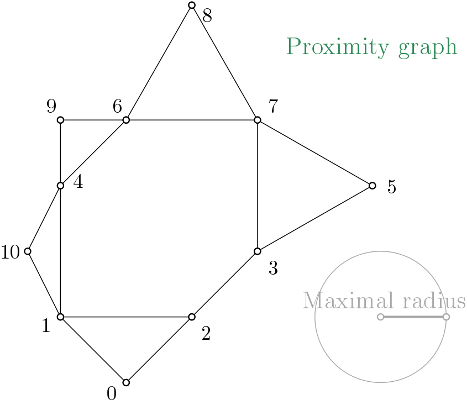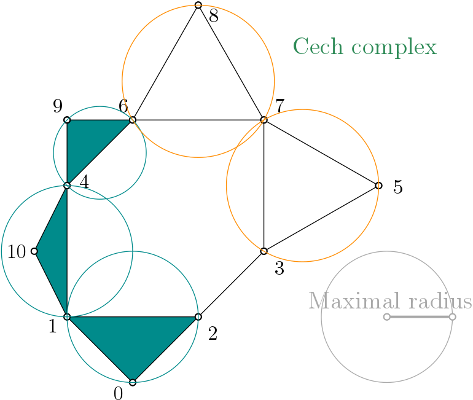Čech complex

## Classes

class  Gudhi::cech_complex::Cech_complex< Kernel, SimplicialComplexForCechComplex >
Cech complex class. More...

# Čech complex definition

Čech complex (Wikipedia) is a simplicial complex constructed from a proximity graph. The set of all simplices is filtered by the radius of their minimal enclosing ball.

The input shall be a range of points where a point is defined as CGAL kernel Point_d.

Remarks
For people only interested in the topology of the Čech complex (for instance persistence), Alpha complex is equivalent to the Čech complex and much smaller if you do not bound the radii. Čech complex can still make sense in higher dimension precisely because you can bound the radii.

## Algorithm

Cech_complex first builds a proximity graph from a point cloud. The filtration value of each edge of the Gudhi::Proximity_graph is computed using CGAL kernel functions.

All edges that have a filtration value strictly greater than a user given maximal radius value, $$max\_radius$$, are not inserted into the complex.

Vertex name correspond to the index of the point in the given range (aka. the point cloud).Čech complex proximity graph representation

When creating a simplicial complex from this proximity graph, Cech_complex inserts the proximity graph into the simplicial complex data structure, and then expands the simplicial complex when required.

On this example, as edges $$(x,y)$$, $$(y,z)$$ and $$(z,y)$$ are in the complex, the minimal ball radius containing the points $$(x,y,z)$$ is computed.

$$(x,y,z)$$ is inserted to the simplicial complex with the filtration value set with $$mini\_ball\_radius(x,y,z))$$ iff $$mini\_ball\_radius(x,y,z)) \leq max\_radius$$.

And so on for higher dimensions.Čech complex expansion

This radius computation is the reason why the Cech_complex is taking much more time to be computed than the Rips complex but it offers more topological guarantees.

## Example from a point cloud

This example builds the proximity graph from the given points, and maximal radius values. Then it creates a Simplex_tree with it.

#include <gudhi/Cech_complex.h>
#include <gudhi/Simplex_tree.h>
#include <CGAL/Epeck_d.h> // For EXACT or SAFE version
#include <iostream>
#include <string>
#include <vector>
int main() {
// Type definitions
using Kernel = CGAL::Epeck_d<CGAL::Dimension_tag<2>>;
using Point = typename Kernel::Point_d;
using Point_cloud = std::vector<Point>;
Point_cloud points;
points.emplace_back(1., 0.); // 0
points.emplace_back(0., 1.); // 1
points.emplace_back(2., 1.); // 2
points.emplace_back(3., 2.); // 3
points.emplace_back(0., 3.); // 4
points.emplace_back(3. + std::sqrt(3.), 3.); // 5
points.emplace_back(1., 4.); // 6
points.emplace_back(3., 4.); // 7
points.emplace_back(2., 4. + std::sqrt(3.)); // 8
points.emplace_back(0., 4.); // 9
points.emplace_back(-0.5, 2.); // 10
// ----------------------------------------------------------------------------
// Init of a Cech complex from points
// ----------------------------------------------------------------------------
Simplex_tree stree;
cech_complex_from_points.create_complex(stree, 2);
// ----------------------------------------------------------------------------
// Display information about the one skeleton Cech complex
// ----------------------------------------------------------------------------
std::clog << "Cech complex is of dimension " << stree.dimension() << " - " << stree.num_simplices() << " simplices - "
<< stree.num_vertices() << " vertices." << std::endl;
std::clog << "Iterator on Cech complex simplices in the filtration order, with [filtration value]:" << std::endl;
for (auto f_simplex : stree.filtration_simplex_range()) {
std::clog << " ( ";
for (auto vertex : stree.simplex_vertex_range(f_simplex)) {
std::clog << vertex << " ";
}
std::clog << ") -> "
<< "[" << stree.filtration(f_simplex) << "] ";
std::clog << std::endl;
}
return 0;
}
Simplex Tree data structure for representing simplicial complexes.
Definition: Simplex_tree.h:79
Options::Filtration_value Filtration_value
Type for the value of the filtration function.
Definition: Simplex_tree.h:86
Filtration_simplex_range const & filtration_simplex_range(Indexing_tag=Indexing_tag())
Returns a range over the simplices of the simplicial complex, in the order of the filtration.
Definition: Simplex_tree.h:271
Simplex_vertex_range simplex_vertex_range(Simplex_handle sh) const
Returns a range over the vertices of a simplex.
Definition: Simplex_tree.h:282
static Filtration_value filtration(Simplex_handle sh)
Returns the filtration value of a simplex.
Definition: Simplex_tree.h:535
size_t num_vertices() const
Returns the number of vertices in the complex.
Definition: Simplex_tree.h:572
int dimension(Simplex_handle sh)
Returns the dimension of a simplex.
Definition: Simplex_tree.h:600
size_t num_simplices()
returns the number of simplices in the simplex_tree.
Definition: Simplex_tree.h:578
Cech complex class.
Definition: Cech_complex.h:43
Value type for a filtration function on a cell complex.
Definition: FiltrationValue.h:20

When launching (maximal enclosing ball radius is 1., is expanded until dimension 2):

\$> ./Cech_complex_example_from_points

the program output is:

Iterator on Cech complex simplices in the filtration order, with [filtration value]:
( 0 ) -> 
( 1 ) -> 
( 2 ) -> 
( 3 ) -> 
( 4 ) -> 
( 5 ) -> 
( 6 ) -> 
( 7 ) -> 
( 8 ) -> 
( 9 ) -> 
( 10 ) -> 
( 9 4 ) -> [0.5]
( 9 6 ) -> [0.5]
( 10 1 ) -> [0.559017]
( 10 4 ) -> [0.559017]
( 1 0 ) -> [0.707107]
( 2 0 ) -> [0.707107]
( 3 2 ) -> [0.707107]
( 6 4 ) -> [0.707107]
( 9 6 4 ) -> [0.707107]
( 2 1 ) -> 
( 2 1 0 ) -> 
( 4 1 ) -> 
( 5 3 ) -> 
( 7 3 ) -> 
( 7 5 ) -> 
( 7 6 ) -> 
( 8 6 ) -> 
( 8 7 ) -> 
( 10 4 1 ) ->